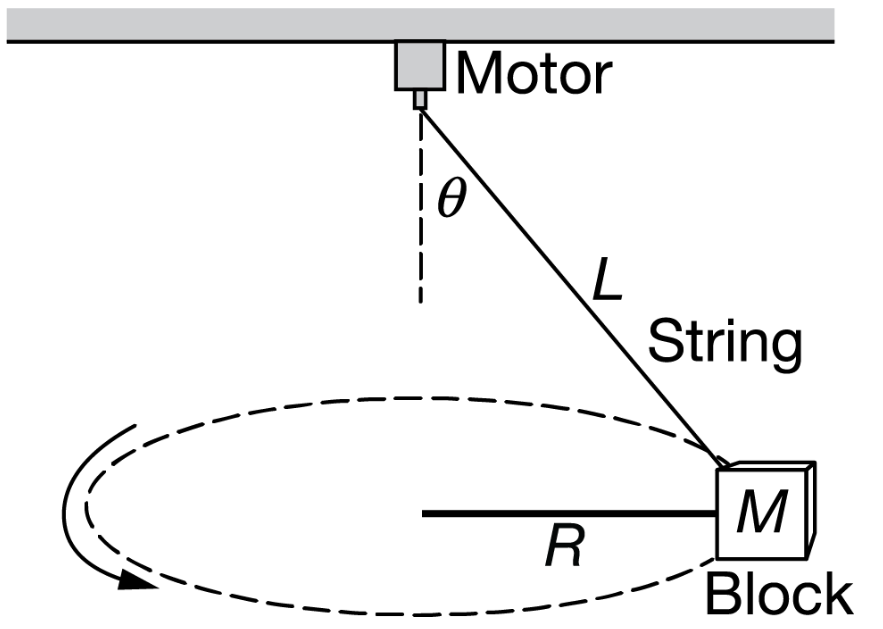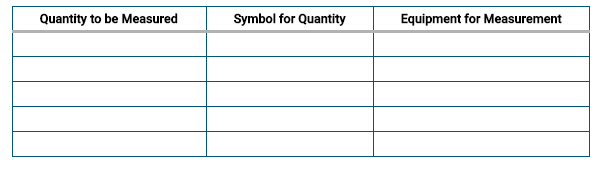# Question Solved1 AnswerThis question is a long free-response question. Show your work for each part of the question. In the apparatus shown above, one end of a string of length L is attached to a block of mass M and the other end is connected to the axle of a motor that rotates, causing the block to move in a circle of radius R at a constant speed vT such that the string makes an angle θ with the vertical. A student wants to use the apparatus to make measurements and create a graph that can be used to estimate the acceleration g due to gravity at the surface of Earth. The student can adjust the motor to achieve different tangential speeds of the block. (b) Using the experimental apparatus shown above and the available equipment, design an experimental procedure the student could use to estimate the acceleration g due to gravity at the surface of Earth. i. In the table below, list the quantities and associated symbols that would be measured in the experiment and the equipment used to measure them. Also list the equipment that would be used to measure each quantity. You do not need to fill in every row. If you need additional rows, you may add them to the space just below the table. ii. Describe an experimental procedure to estimate the acceleration g due to gravity at the surface of Earth using the apparatus. Give enough detail so that another student could replicate the experiment. As needed, include a diagram of the experimental setup. Assume equipment usually found in a school physics laboratory is available. V Motor String -RM --- Block Quantity to be Measured | Symbol for Quantity Equipment for MeasurementThis question is a long free-response question. Show your work for each part of the question.In the apparatus shown above, one end of a string of length L is attached to a block of mass M and the other end is connected to the axle of a motor that rotates, causing the block to move in a circle of radius R at a constant speed vT such that the string makes an angle θ with the vertical. A student wants to use the apparatus to make measurements and create a graph that can be used to estimate the acceleration g due to gravity at the surface of Earth. The student can adjust the motor to achieve different tangential speeds of the block.

(b) Using the experimental apparatus shown above and the available equipment, design an experimental procedure the student could use to estimate the acceleration g due to gravity at the surface of Earth.

i. In the table below, list the quantities and associated symbols that would be measured in the experiment and the equipment used to measure them. Also list the equipment that would be used to measure each quantity. You do not need to fill in every row. If you need additional rows, you may add them to the space just below the table.ii. Describe an experimental procedure to estimate the acceleration g due to gravity at the surface of Earth using the apparatus. Give enough detail so that another student could replicate the experiment. As needed, include a diagram of the experimental setup. Assume equipment usually found in a school physics laboratory is available.

Transcribed Image Text: V Motor String -RM --- Block Quantity to be Measured | Symbol for Quantity Equipment for Measurement
More
Transcribed Image Text: V Motor String -RM --- Block Quantity to be Measured | Symbol for Quantity Equipment for Measurement